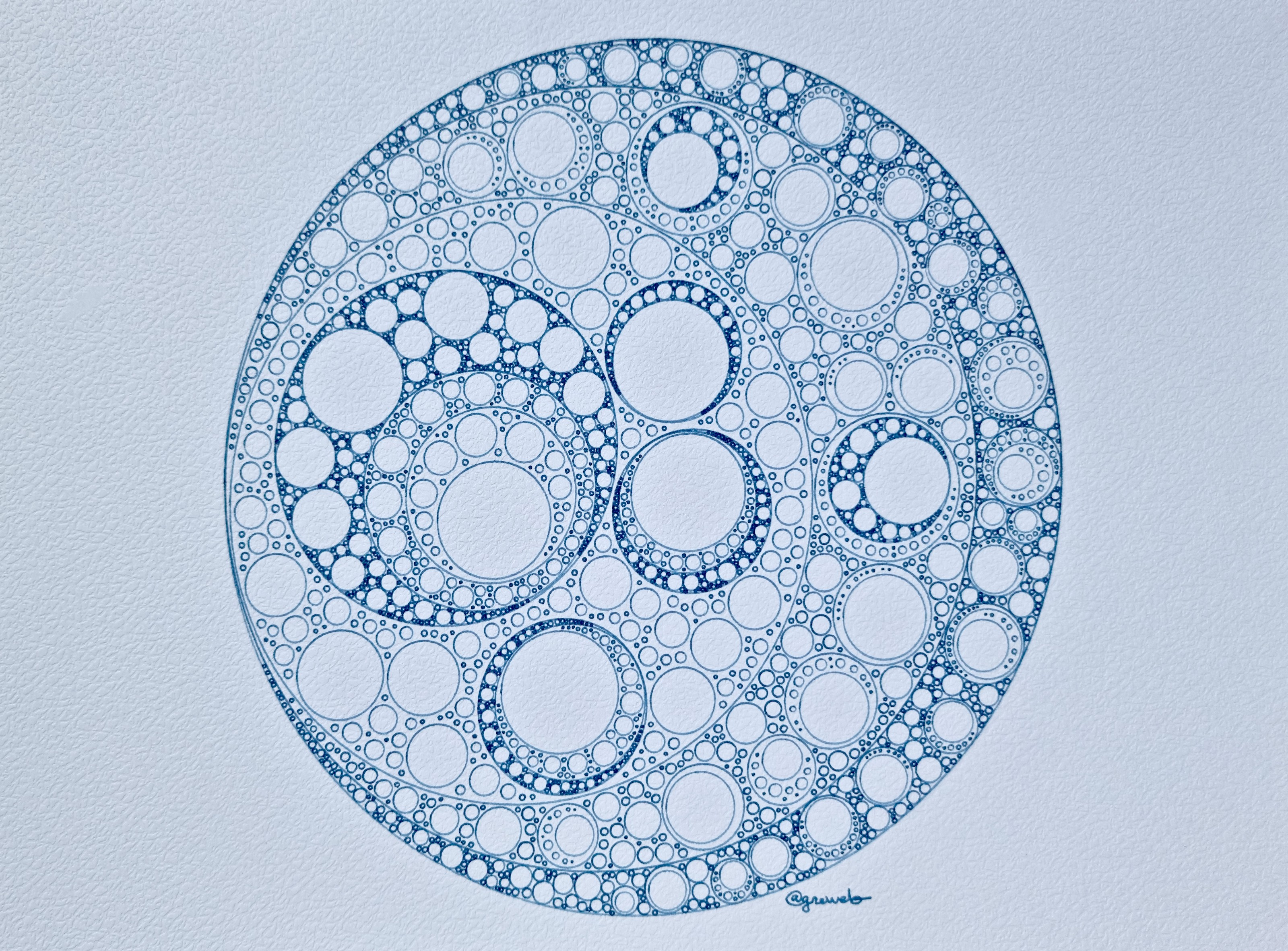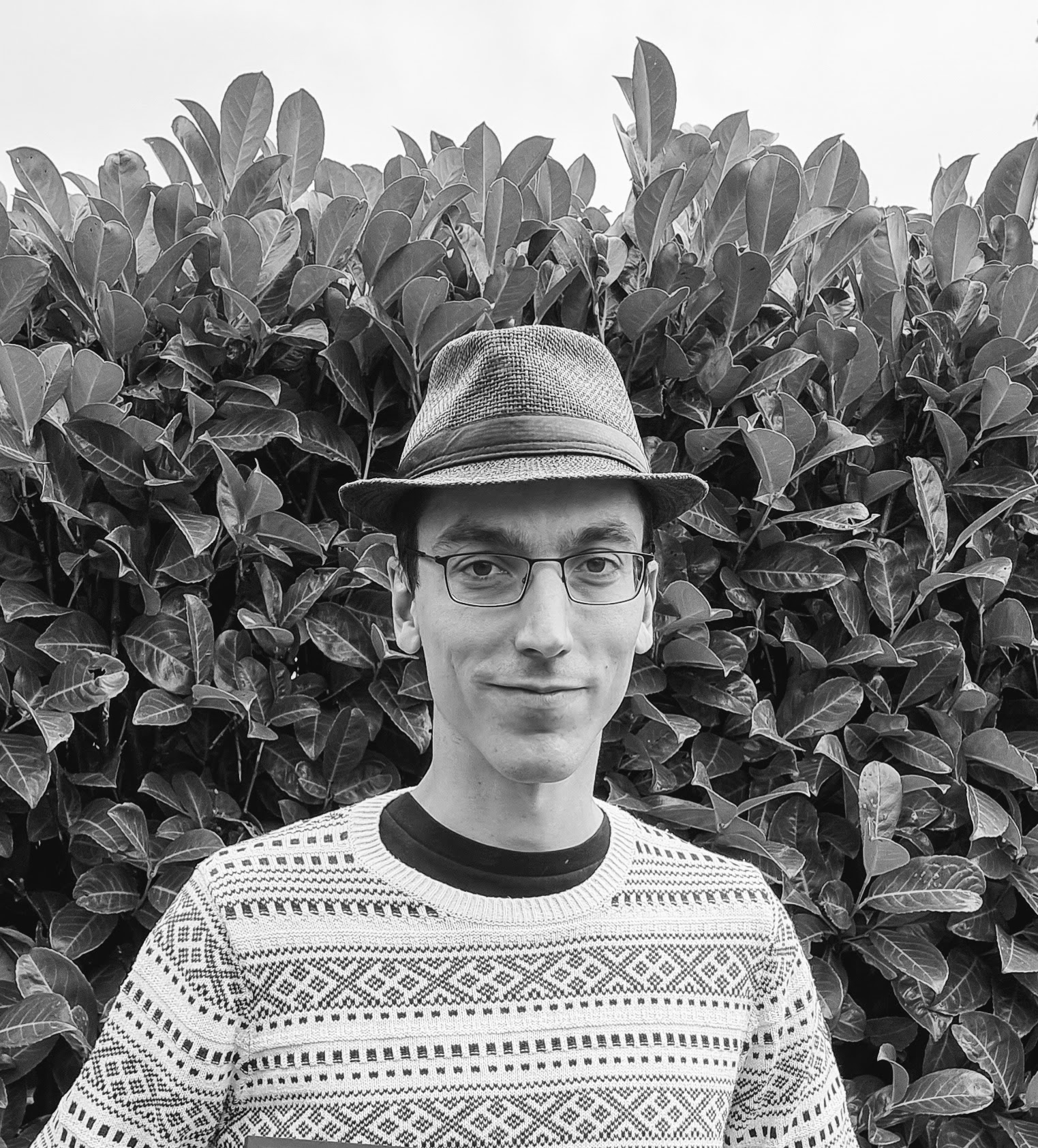# greweb.me / Plot #203 "circles recursion & ordered noise"As you may notice, I like randomness a lot. Exploring the beauty of noise is also recreating Nature's pattern and trying to put back some order in that randomness.

This creation is an unusual version of "circle packing" that instead of a pure random distribution will run a few times to actually try to find the biggest circle to place. This makes the distribution less random and way more ordered. However, the fact there is a "a few times" maximum tries gives some room for some randomness and not "pure order".

The algorithm at stake is relatively simple, I will have to improve it in future to parallelize the computation.

``````fn packing(
seed: f64,
iterations: usize,
desired_count: usize,
optimize_size: usize, // <--- number of retries to optimize the size
container: &VCircle,
min_scale: f64,
max_scale: f64,
) -> Vec<VCircle> {
let mut circles = Vec::new();
let mut tries = Vec::new();
let mut rng = rng_from_seed(seed);
let x1 = container.x - container.r;
let y1 = container.y - container.r;
let x2 = container.x + container.r;
let y2 = container.y + container.r;
let max_scale = max_scale.min(container.r);
for _i in 0..iterations {
let x: f64 = rng.gen_range(x1, x2);
let y: f64 = rng.gen_range(y1, y2);
if let Some(size) = search_circle_radius(&container, &circles, x, y, min_scale, max_scale) {
let circle = VCircle::new(x, y, size - pad);
// innovation happens here, basically we keep pushing until we reach the nb
tries.push(circle);
if tries.len() > optimize_size {
// then we grab the biggest
tries.sort_by(|a, b| b.r.partial_cmp(&a.r).unwrap());
let c = tries;
circles.push(c.clone());
tries = Vec::new();
}
}
if circles.len() > desired_count {
break;
}
}
circles
}
``````creative coder experimenting with GLSL shaders, Rust, and fountain pens robot plots. infinite noise explorer.